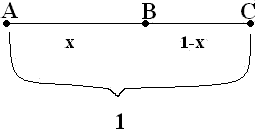Quandaries and Queries hi, my name is cristina and im a middle school student. im having trouble with golden ratios. i know what it is but im doing a project and nowhere on the internat have i found an answer to my question..... please help.... here is the question:For the above segment, let x represent the longer segment. to find the golden ratio, write a proportion such that the longer of the two segments is the geometric mean between the shorter segment and the entire segment.Use the quadratic Formula to solve the proportion for X. Find the value in both radical and decimal form. please answer this question for me or explain to me how to do it! im stressing. its a major project and this its the last question i need to answer. please help? thanks.                                                                                   sincearly, cristina Cristina, what you have is a poor way of describing the Golden ratio. "The longer of the two segments is the geometric mean between the shorter segment and the entire segment" says that x = squareroot((1-x)/1) which can be converted to x2 = 1-x or x2+x-1 = 0 and the golden ration is the larger root of this quadratic (use the quadratic formula). A proper way to describe the golden ratio from your diagram is that x and 1-x and 1 satisfy that the proportions of (1-x)/x and x/1 are the same, which leads to x2 = 1-x as above, etc. Penny Go to Math Central